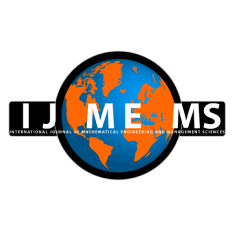### International Journal of Mathematical, Engineering and Management Sciences

#### ISSN: 2455-7749 . Open Access

On Solving Bi-level Multi-Objective Fully Quadratic Fractional Optimization Model with Fuzzy Demands

#### On Solving Bi-level Multi-Objective Fully Quadratic Fractional Optimization Model with Fuzzy Demands

Namrata Rani
Department of Mathematics, Maharishi Markandeshwar (Deemed to be University), Mullana-Ambala, India.

Vandana Goyal
Department of Mathematics, Maharishi Markandeshwar (Deemed to be University), Mullana-Ambala, India.

Deepak Gupta
Department of Mathematics, Maharishi Markandeshwar (Deemed to be University), Mullana-Ambala, India.

;
Accepted on October 19, 2021

Abstract

This paper has been designed to introduce the method for solving the Bi-level Multi-objective (BL-MO) Fully Quadratic Fractional Optimization Model through Fuzzy Goal Programming (FGP) approach by utilising non-linear programming. In Fully Quadratic Fractional Optimization Model, the objective functions are in fractional form, having quadratic functions in both numerator and denominator subject to quadratic constraints set. The motive behind this paper is to provide a solution to solve the BL-MO optimization model in which number of decision-makers (DM) exists at two levels in the hierarchy. First, the fractional functions with fuzzy demand, which are in the form of fuzzy numbers, are converted into crisp models by applying the concept of α-cuts. After that, membership functions are developed which are corresponding to each decision-maker’s objective and converted into simpler form to avoid complications due to calculations. Finally, the model is simplified by applying FGP approach, and a compromised solution to the initial model is obtained. An algorithm, flowchart and example are also given at the end to explain the study of the proposed model.

Keywords- α-cut set, BL-MO optimization model, Fuzzy programming, Fully quadratic fractional programming.

Citation

Rani, N., Goyal, V., & Gupta, D. (2021). On Solving Bi-level Multi-Objective Fully Quadratic Fractional Optimization Model with Fuzzy Demands. International Journal of Mathematical, Engineering and Management Sciences, 6(6), 1612-1628. https://doi.org/10.33889/IJMEMS.2021.6.6.096.